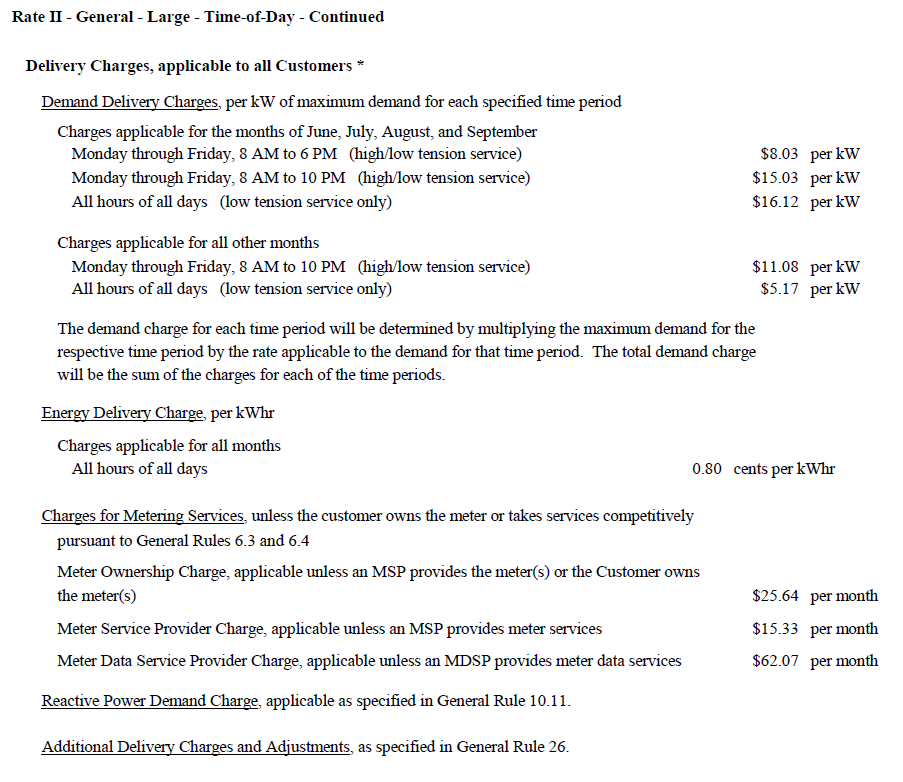Question-and-Answer Resource for the Building Energy Modeling Community
Get s tarted with the Help page

# Defining demand charge for three block rates

I am trying to define the tariff for the time-of-day rates defined as -1. I am trying to use the three blocks applicable at the same time, i.e., for peak hour in June is it going to be (8.03+15.03+16.12)? This is for each 1/2 hour max demand, isn't this too high? The last rate(16.12) says 'low tension only'? Is this correct understanding? While other months the max is (5.17+11.08) for demand charges.
2. How to define the TimeOfUseSchedule for the common interval of 8 am to 6 pm (as peak(1) and mid peak(4))? (EnergyCharges is small at $0.008 with no peak rates.) 3. How will the off-peak (3) for winter and summer be aggregated ? Only one variable is automatically generated for 'offPeakDemand'. edit retag close merge delete ## 1 Answer Sort by » oldest newest most voted 1. In my interpretation of the rate, if the peak demand was in June at 2pm on a Wednesday, and low-tension service is being used, then the demand charge would be (8.03 + 15.03 + 16.12) times the peak demand. If the peak demand for January was set at 2pm on a Wednesday and low-tension service is being used, then the demand charge would be (11.08 + 5.17 ) times the peak demand. It is important to understand the timing of the peak demand and if the monthly peak does not occur during 8am to 6pm, then the peak during that particular period needs to be used for computing that portion of the demand charge and the same goes for 8am to 10pm. 2. The Time of Use Period Schedule Name field should identify a schedule that for the summer months uses 1 for the 8am to 6pm times to represent the peak hours, 2 for the 6pm to 10pm times to represent the shoulder hours and 3 all other hours. Then use UtilityCost:Charge:Simple of 8.03 with a Source Variable of PeakDemand and another UtilityCost:Simple of 15.03 with a Source Variable of PeakAndShoulderDemand and a third UtilityCost:Charge:Simple of 16.12 with a source Variable of TotalDemand. The energy cost would be computed using a UtilityCost:Charge:Simple of 0.0080 with a source variable of TotalEnergy. A similar approach would be used for the winter demand charges. 3. I don't think you will need the offPeakDemand variable. more ## Comments Thanks @JasonGlazer 1. The way I understand from your reply is that the demand charges will be for the block with maximum (maxDemand)(rates applicable). If it does not occur during peak-hours, then only the off-peak rate will be applied as MaxDemand (11.08+5.17) and the (8.03 + 15.03 + 16.12) rate will not even be used. No, that is not quite it. Lets say the peak demand is at 8pm at 500 kW but for the times between 8am and 6pm the demand reaches 400 kW at 4pm you would multiply the 500 kW for the 8.03 + 15.03 but you would multiply 400 kW times 16.12 to capture that portion of the demand charges. Thanks @JasonGlazer, In your example, what values the variables will have for 8 pm( 0,400,500), (peakDemand, peakAndSholder, totalDemand). When do they reset? Is the$ for demand calculated every 1/2 hour and then move to next calculation of dollar or once in a month?

1

In my example the 8pm demand would be for 500 kW. The demand is the maximum amount of kW (in your case averaged over a 1/2 hour) but the demand charge is just calculated once for each bill (usually once per month).